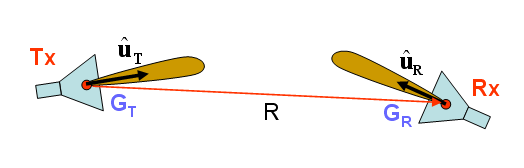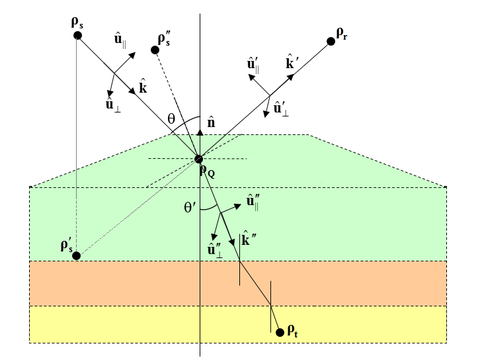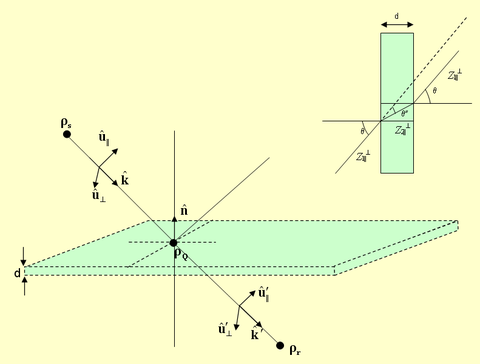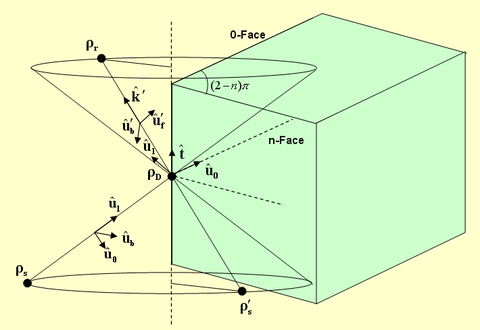# SBR Method

## Free-Space Wave Propagation

In a free-space line-of-sight (LOS) communication system, the signal propagates directly from the transmitter to the receiver without encountering any obstacles (scatterers). Electromagnetic waves propagate in the form of spherical waves with a functional dependence of ej(ωt-k0R)/R, where R is the distance between the transmitter and receiver, $\omega = 2\pi f$, f is the signal frequency, $k_0 = \frac{\omega}{c} = \frac{2\pi}{\lambda}$, c is the speed of light, and λ0 is the free-space wavelength at the operational frequency. By the time the signal arrives at the location of the receiver, it undergoes two changes. It is attenuated and its power drops by a factor of 1/R2, and additionally, it experiences a phase shift of $\frac{2\pi R}{\lambda_0}$, which is equivalent to a time delay of R/c. The signal attenuation from the transmitter to the receiver is usually quantified by Path Loss defined as the ratio of the received signal power (PR) to the transmitted signal power (PT). Assuming isotropic transmitting and receiving radiators (i.e. radiating uniformly in all directions), the Path Loss in a free-space line-of-sight communication system is given by Friis’ formula:

$\frac{P_R}{P_T} = \left( \frac{\lambda_0}{4\pi R} \right)^2$

The above formula assumes that the receiving antenna is polarization-matched. Normally, there is a polarization mismatch between the transmitting and receiving antennas. In the case of directional transmitting and receiving antennas, Friis’ formula takes the following form:

$P_R = P_T \, G_T G_R \left( \frac{\lambda_0}{4\pi R} \right)^2 \left| \mathbf{ \hat{u}_T \cdot \hat{u}_R } \right|^2$

where uT and uR are the unit polarization vectors of the transmitting and receiving antennas, and GT and GR are their gains, respectively.A line-of-sight (LOS) propagation scenario.

## Basic Wave Interaction Mechanisms

EM.Terrano discretizes all of the objects in your propagation scene into flat triangular facets. Obviously, rectangular and cubic objects preserve their geometric shapes through this discretization. Objects with curved surfaces such as cylinders, cones or spheres, are approximated by triangular surface mesh representations. The geometric fidelity of the resulting mesh depends on the specified mesh edge length. When a ray hits a triangular facet, the propagating spherical wave is approximated as a plane wave at the specular point. The reflection and transmission coefficients of the surface are calculated at the operational frequency and at the particular ray incident angle.

A new reflected ray is generated at the specular point, which starts traveling and bouncing around in the scene. If the obstructing surface is penetrable, a second transmitted ray is generated and added to the scene. If the ray hits the edge of an obstacle, it is diffracted from that edge. This leads to the creation of a cone of new rays, which greatly complicate the computational problem. The Uniform Theory of Diffraction (UTD) is used to calculate the wedge diffraction coefficients at the edges of scattering blocks. Note that reflection, transmission and diffraction coefficients are all dependent on the polarization of the incident plane wave.

A receiver may receive a large number of rays: direct line-of-sight rays from the transmitter, rays reflected or diffracted off the ground or terrain, rays reflected or diffracted from buildings or rays transmitted through buildings. Each received ray is characterized by its power, delay and angles of arrival, which are the spherical coordinate angles θ and φ of the incoming ray. The actual signal received and detected by the receiver is the superposition of all these rays with different power levels and different time delays. Most of the time, you will be interested in the coverage map of an area, which shows how much power is received by a grid of receivers spread over the area from a given fixed transmitter.

## Ray Reflection & Transmission

The incident, reflected and transmitted rays are each characterized by a triplet of unit vectors:

• $( \mathbf{ \hat{u}_{\|}, \hat{u}_{\perp}, \hat{k} } )$ representing the incident parallel polarization vector, incident perpendicular polarization vector and incident propagation vector, respectively.
• $( \mathbf{ \hat{u}_{\|}^{\prime}, \hat{u}_{\perp}', \hat{k}' } )$ representing the reflected parallel polarization vector, reflected perpendicular polarization vector and reflected propagation vector, respectively.
• $( \mathbf{ \hat{u}_{\|}^{\prime\prime}, \hat{u}_{\perp}^{\prime\prime}, \hat{k}^{\prime\prime} } )$ representing the transmitted parallel polarization vector, transmitted perpendicular polarization vector and transmitted propagation vector, respectively.The incident, reflected and transmitted rays at the interface between two dielectric media.

The reflected ray is assumed to originate from a virtual image source point. The three triplets constitute three orthonormal basis systems. Below, it is assumed that the two dielectric media have permittivities ε1 and ε2, and permeabilities μ1 and μ2, respectively. A lossy medium with a conductivity σ can be modeled by a complex permittivity εr = ε'r –jσ/ε0. Assuming n to be the unit normal to the interface plane between the two media, and Z0 = 120Ω , the incident polarization vectors as well as all the reflected and transmitted vectors are found as:

$\mathbf{ \hat{u}_{\perp} = \frac{\hat{k} \times \hat{n}}{|\hat{k} \times \hat{n}|} }$
$\mathbf{ \hat{u}_{\|} = \hat{u}_{\perp} \times \hat{k} }$

The reflected unit vectors are found as:

$\mathbf{ \hat{k}' = \hat{k} - 2(\hat{k} \cdot \hat{n}) \hat{n} }$
$\mathbf{ \hat{u}_{\perp}' = \hat{u}_{\perp} }$
$\mathbf{ \hat{u}_{\|}' = \hat{u}_{\perp}' \times \hat{k}' }$

The transmitted unit vectors are found as:

$\mathbf{ \hat{k}^{\prime\prime} = \hat{n} \times a - \sqrt{1-a \cdot a} \; \hat{n} }$
$\mathbf{ \hat{u}_{\perp}^{\prime\prime} = \hat{u}_{\perp} }$
$\mathbf{ \hat{u}_{\|}^{\prime\prime} = \hat{u}_{\perp}^{\prime\prime} \times \hat{k}^{\prime\prime} }$

where

$\mathbf{a} = (k_1/k_2) \mathbf{\hat{k} \times \hat{n}}$
$k_1 = k_0 \sqrt{\varepsilon_1 \mu_1}$
$k_2 = k_0 \sqrt{\varepsilon_2 \mu_2}$
$\eta_1 = Z_0 \sqrt{\mu_1 / \varepsilon_1}$
$\eta_2 = Z_0 \sqrt{\mu_2 / \varepsilon_2}$

$\sin\theta^{\prime\prime} = \frac{k_1}{k_2}\sin\theta \text{ if } \sin\theta \le k_2/k_1$

The reflection coefficients at the interface are calculated for the two parallel and perpendicular polarizations as:

$R_{\|} = \frac { \eta_2(\mathbf{ \hat{k}^{\prime\prime} \cdot \hat{n} }) - \eta_1(\mathbf{ \hat{k} \cdot \hat{n} }) } { \eta_2(\mathbf{ \hat{k}^{\prime\prime} \cdot \hat{n} }) + \eta_1(\mathbf{ \hat{k} \cdot \hat{n} }) } = \frac{\eta_2 \cos\theta^{\prime\prime} - \eta_1 \cos\theta} {\eta_2 \cos\theta^{\prime\prime} + \eta_1 \cos\theta} = \frac{Z_{2\|} - Z_{1\|}} {Z_{2\|} + Z_{1\|}}$

$R_{\perp} = \frac { \eta_2(\mathbf{ \hat{k} \cdot \hat{n} }) - \eta_1(\mathbf{ \hat{k}^{\prime\prime} \cdot \hat{n} }) } { \eta_2(\mathbf{ \hat{k} \cdot \hat{n} }) + \eta_1(\mathbf{ \hat{k}^{\prime\prime} \cdot \hat{n} }) } = \frac{\eta_2 / \cos\theta^{\prime\prime} - \eta_1 / \cos\theta} {\eta_2 / \cos\theta^{\prime\prime} + \eta_1 / \cos\theta} = \frac{Z_{2\perp} - Z_{1\perp}} {Z_{2\perp} + Z_{1\perp}}$

## Penetration through Thin Walls or Surfaces

In "Thin Wall Approximation", we assume that an incident ray gives rise to two rays, one is reflected at the specular point, and the other is transmitted almost in the same direction as the incident ray. The reflected ray is assumed to originate from a virtual image source point. Similar to the case of reflection and transmission at the interface between two dielectric media, here too we have three triplets of unit vectors, which all form orthonormal basis systems.The incident and transmitted rays through a thin wall.

The transmission coefficients are calculated for the two parallel and perpendicular polarizations as:

$T_{\|} = \frac{(1-{\Gamma_{\|}}^2) \exp(-jk_2 d (\mathbf{ \hat{k}^{\prime\prime} \cdot \hat{n}}))} { 1-{\Gamma_{\|}}^2 \exp( -2jk_2 d (\mathbf{ \hat{k}^{\prime\prime} \cdot \hat{n} }) ) }$

$T_{\perp} = \frac{(1-{\Gamma_{\perp}}^2) \exp(-jk_2 d (\mathbf{ \hat{k}^{\prime\prime} \cdot \hat{n}}))} { 1-{\Gamma_{\perp}}^2 \exp( -2jk_2 d (\mathbf{ \hat{k}^{\prime\prime} \cdot \hat{n} }) ) }$

where

$\Gamma_{\|} = \frac{ \eta_2(\mathbf{ \hat{k}^{\prime\prime} \cdot \hat{n} }) - \eta_1(\mathbf{ \hat{k} \cdot \hat{n} }) } { \eta_2(\mathbf{ \hat{k}^{\prime\prime} \cdot \hat{n} }) + \eta_1(\mathbf{ \hat{k} \cdot \hat{n} }) } = \frac{\eta_2 \cos\theta^{\prime\prime} - \eta_1 \cos\theta} {\eta_2 \cos\theta^{\prime\prime} + \eta_1 \cos\theta} = \frac{Z_{2\|} - Z_{1\|}} {Z_{2\|} + Z_{1\|}}$

$\Gamma_{\perp} = \frac{ \eta_2(\mathbf{ \hat{k} \cdot \hat{n} }) - \eta_1(\mathbf{ \hat{k}^{\prime\prime} \cdot \hat{n} }) } { \eta_2(\mathbf{ \hat{k} \cdot \hat{n} }) + \eta_1(\mathbf{ \hat{k}^{\prime\prime} \cdot \hat{n} }) } = \frac{\eta_2 / \cos\theta^{\prime\prime} - \eta_1 / \cos\theta} {\eta_2 / \cos\theta^{\prime\prime} + \eta_1 / \cos\theta} = \frac{Z_{2\perp} - Z_{1\perp}} {Z_{2\perp} + Z_{1\perp}}$

## Wedge Diffraction from Edges

For the purpose of calculation of diffraction from building edges, we define a "Wedge" as having two faces, the 0-face and the n-face. The wedge angle is a = (2-n)p, where the parameter n is required for the calculation of diffraction coefficients. All the diffracted rays lie on a cone with its vertex at the diffraction point and a wedge angle equal to the angle of incidence in the opposite direction. A diffracted ray is assumed to originate from a virtual image source point. Three triplets of unit vectors are defined as follows:

• $\mathbf{(\hat{u}_0, \hat{u}_l, \hat{t})}$ representing the unit vector normal to the edge and lying in the plane of the 0-face, the unit vector normal to the 0-face, and the unit vector along the edge, respectively.
• $\mathbf{(\hat{u}_f, \hat{u}_b, \hat{t})}$ representing the incident forward polarization vector, incident backward polarization vector and incident propagation vector, respectively.
• $\mathbf{(\hat{u}_f', \hat{u}_b', \hat{t}')}$ representing the diffracted forward polarization vector, diffracted backward polarization vector and diffracted propagation vector, respectively.The incident ray and diffracted ray cone at the edge of a building.

The three triplets constitute three orthonormal basis systems. The propagation vector k' of the diffracted ray has to be constructed based on the diffraction cone as follows:

$\mathbf{\hat{k}'} = \cos\phi_w \mathbf{\hat{u}_0} + \sin\phi_w \mathbf{\hat{u}_l} + \mathbf{(\hat{k} \cdot \hat{t}) \hat{t}}, \quad 0 \le \phi_w \le \alpha$

where the resolution of the angle θw is chosen to be the same as the resolution of the incident ray.

The other unit vectors for the incident and diffracted rays are found as:

$\mathbf{ \hat{u}_f = \frac{\hat{k} \times \hat{t}}{|\hat{k} \times \hat{t}|} }$
$\mathbf{ \hat{u}_b = \hat{k} \times \hat{u}_f }$
$\mathbf{ \hat{u}_f' = \frac{\hat{k}' \times \hat{t}}{|\hat{k}' \times \hat{t}|} }$
$\mathbf{ \hat{u}_b' = \hat{k}' \times \hat{u}_f' }$

The diffraction coefficients are calculated in the following way:

D_s = \frac{-e^{-j\pi/4}}{2n \sqrt{2\pi k} \sin\beta_0'} \left\lbrace \begin{align} & \cot \left(\frac{\pi + (\phi-\phi')}{2n}\right) F[kLa^+(\phi-\phi')] + \cot \left(\frac{\pi - (\phi-\phi')}{2n}\right) F[kLa^-(\phi-\phi')] + \\ & R_{0 \perp} \cot \left(\frac{\pi - (\phi+\phi')}{2n}\right) F[kLa^-(\phi+\phi')] + R_{n \perp} \cot \left(\frac{\pi + (\phi+\phi')}{2n}\right) F[kLa^+(\phi+\phi')] \end{align} \right\rbrace

D_h = \frac{-e^{-j\pi/4}}{2n \sqrt{2\pi k} \sin\beta_0'} \left\lbrace \begin{align} & \cot \left(\frac{\pi + (\phi-\phi')}{2n}\right) F[kLa^+(\phi-\phi')] + \cot \left(\frac{\pi - (\phi-\phi')}{2n}\right) F[kLa^-(\phi-\phi')] + \\ & R_{0 \|} \cot \left(\frac{\pi - (\phi+\phi')}{2n}\right) F[kLa^-(\phi+\phi')] + R_{n \|} \cot \left(\frac{\pi + (\phi+\phi')}{2n}\right) F[kLa^+(\phi+\phi')] \end{align} \right\rbrace

where F(x) is the Fresnel Transition function:

$F(x) = 2j \sqrt{x} e^{jx} \int_{\sqrt{x}}^{\infty} e^{-j\tau^2} \, d\tau$

In the above equations, we have

\begin{align} s = |\rho_D - \rho_S| \\ s' = |\rho_D - \rho_r| \end{align}
$L = \frac{s s' \sin^2 \beta'}{s + s'}$
$a^{\pm}(\nu) = 2\cos^2 \left( \frac{2n\pi N^{\pm} - \nu}{2} \right), \quad \nu = \phi \pm \phi'$

where $N^{\pm}$ are the integers which most closely satisfy the equations $2n\pi N^{\pm} - \nu = \pm \pi$.